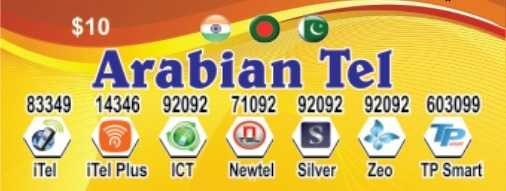3 years ago ·Translate

Rate Update-

8801=240 min
0091=690 min

Probashi Card

BD Gery 8801= 240 min
BD White- 008801= 104 min

India::

91= 504 min
091= 1244 min
0091=690 min

Pakistan Cli::

9230= 69 min
9231= 96 min
9232= 62 min
9233= 63 min
9234= 74 min

Pakistan Gery-009230=150 Min
Pakistan Gery-009231=200 Min
Pakistan Gery-009232=153 Min
Pakistan Gery-009233=163 Min
Pakistan Gery-009234= 142 min

Bdt- 140 tk

কার্ডের ব্যবস্হা আছে।

ডুবাই, সৌদি, ওমান,কাতার,
কুয়েত,বাংলাদেশের।।